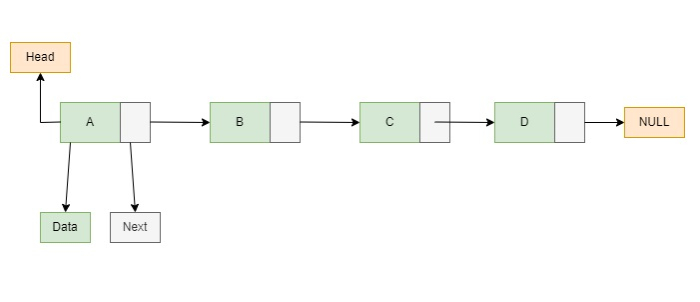# Linked List representation in Javascript

Linked List is an ordered collection of data elements. In linked list the data will be represented in Nodes. Node has two parts, the first part will be holding the data of the element and second part of the node (pointer) will store the address of the very next node. In linked list elements are stored in a sequential manner.There are several operations in Linked list, which are adding a node, deleting a node and searching a node. which are detailed in the below scenarios.

### Creating a Node

Let’s see the scenario of linked list node below −

//Creating a node
class Node {
constructor(element){
this.element = element,
this.next = null // next of the node will be NULL.
}
}


In the above scenario, we have created a Node which has two properties: element and next. Element holds the data of the node and the second property "next" will holds the pointer referring to the next node. In above example next is referring to NULL because there is only node in linked list.

### Creating a Linked list class

Below is the method to create a linked list class −

class LinkedList{
//Creates a linkedlist with passed element.
constructor(element){
element: element,
next : null
};
this.length = 1;
}
}


In the above snippet, we have created a linked list class that will perform adding, deleting and searching the nodes. And the length of this list is defined as 1, as there is only one node in the linked list (head is also the last node).

• Create a New node by creating instance of node class.
• Point New node’s next to the head.
• Now, make New node as head.

The following is the method to add a node at head position −

//ADDING node at head position in Linkedlist

//Creating New node
const newNode = new Node(element);

//Points this node's next to the head of next node

//Make this node as Head node

//Increase the length
this.length++;
return this;
}


### Deleting a node at Beginning

Following is the algorithm to delete node at head of the Linked List −

• Make the very next element of deleted element as head.
• Now, decrease the length of Linked list by 1.

This is the method below to delete a node at head position −

delete_head_node(){

//removing the node
this.length--;
}


Here, we are performing to delete the element at head position in linked list. The next node of deleted element will become the new head and length of the linked list will reduce by 1.

### Searching an element

Following is the algorithm to search for an element in the Linked List −

• Now, Compare the values
• Return true, if found
• Else, return false.

The below scenario is searching an element in the linked list −

search_Node(element){
//TRAVERSE the list and compare the values

while(curr_Node !== null){
if(curr_Node.element === element)
//returns true if value is present
return true;
curr_Node = curr_Node.next;
}
return false;
}


We are implementing a Linked List data structure in JavaScript. In Linked List we can perform the operations like adding and deleting the elements, searching an element. In the code below we have implemented all the above mentioned operations.

### Example

Let’s look into the final execution of code below −

<!DOCTYPE html>
<html>
<script>
class Node {
constructor(element) {
this.element = element,
this.next = null
}
}
//Creates a linkedlist with passed element.
constructor(element) {
element: element, next: null
};
this.length = 1;
}
const newNode = new Node(element);
this.length++;
return this;
}
// DELETING A NODE
this.length--;
}
//SEARCHING A NODE
search_Node(element) {
while (curr_Node !== null) {
if (curr_Node.element === element)
return true;
curr_Node = curr_Node.next;
}
return false;
}
let getArrayList = [];
while (curr_Node !== null) {
getArrayList.push(curr_Node.element);
curr_Node = curr_Node.next;
}
return getArrayList.join(' → ');
}
}
document.write("Creating a LL with node 44:");
document.write("<br>");
document.write("<br>");

document.write("<br>");
document.write("<br>");

//Deleting
document.write('Deleting (32) node at head position :');
document.write("<br>");
document.write("<br>");

//Searching
document.write('Searching for element 11 :');
document.write("<br>");
document.write("<br>");
document.write("<br>");
document.write('Searching for element 55 :');
document.write("<br>");
document.write("<br>");
</script>
</html>


### Output

The output of the above script will be −

Creating a LL with node 44:
44
33 → 22 → 11 → 44
Deleting (32) node at head position :
22 → 11 → 44
Searching for element 11 :
22 → 11 → 44
true
Searching for element 55 :
22 → 11 → 44
false


Updated on: 18-Nov-2022

142 Views